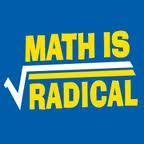# Math

## PROBLEM SOLVING STRATEGIES

• Guess and Test
• Use a Variable
• Draw a Picture
• Look for a Pattern
• Make a List (Tables)
• Solve a Simpler Problem
• Work Backwards
• Make a Model (use objects)
• Solve an Equation
• Use Cases
• Use Direct Reasoning
• Use Indirect Reasoning

## COMMON CORE MATH DOMAINS

The following Common Core math conceptual categories are addressed in 9 - 12th grade.

​Algebra:

• Relationships between Quantities and Reasoning with Equations
• Linear and Exponential Relationships
• Descriptive Statistics
• Expressions and Equations

Geometry:

• Congruence
• Similarity
• Expressing Geometric Properties with Equations
• Similarity Right Triangles and Trigonometry; Geometric Measurements and Dimensions; Conditional Probability and the Rules of Probability

Algebra 2:

• Model and Reason with Equations and Inequalities
• Structure in Expressions and Aritmetic with Polynomials
• Functions
• Geometry and Trigonometry
• Statistics and Probability

Pre Calculus (Math Analysis):

• Algebra, Number Systems, and Vectors
• Functions
• Trigonometry
• Matrices and Conic Systems

For detailed Common Core Math Standards visit: http://achieve.lausd.net/ccss

## AEE MATH TEACHERS

 Ritu Gupta Algebra 1 Marco Rocha Algebra 2 Precalculus Morgan McMurray Geometry

## MATH COURSES OFFERED AT AEE

CC Algebra 1

CC Geometry

CC Geometry Honors

CC Algebra 2

CC Algebra 2 Honors

Precalculus AB

100%-90%             A

89%-80%               B

79%-70%               C

69%-60%               D

59% and below     Fail## COMMON CORE STANDARDS FOR MATHEMATICAL PRACTICES

1. Make sense of problems and persevere in solving them.

2. Reason abstractly and quantitatively.

3. Construct viable arguments and critique the reasoning of others.

4. Model with mathematics.

5. Use appropriate tools strategically.

6. Attend to precision.

7. Look for and make use of structure.

8. Look for and express regularity in repeated reasoning.